# Plotting MCMC draws using the bayesplot package

## Introduction

This vignette focuses on plotting parameter estimates from MCMC draws. MCMC diagnostic plots are covered in the separate vignette Visual MCMC diagnostics, and graphical posterior predictive model checking is covered in the vignette Graphical posterior predictive checks.

### Setup

• ggplot2, in case we want to customize the ggplot objects created by bayesplot
• rstanarm, for fitting the example models used throughout the vignette
``````library("bayesplot")
library("ggplot2")
library("rstanarm")      ``````

### Example model

The bayesplot package provides various plotting functions for visualizing Markov chain Monte Carlo (MCMC) draws from the posterior distribution of the parameters of a Bayesian model.

In this vignette we’ll use draws obtained using the `stan_glm` function in the rstanarm package (Gabry and Goodrich, 2017), but MCMC draws from using any package can be used with the functions in the bayesplot package. See, for example, brms, which, like rstanarm, calls the rstan package internally to use Stan’s MCMC sampler.

``head(mtcars) # see help("mtcars")``
``````                   mpg cyl disp  hp drat    wt  qsec vs am gear carb
Mazda RX4         21.0   6  160 110 3.90 2.620 16.46  0  1    4    4
Mazda RX4 Wag     21.0   6  160 110 3.90 2.875 17.02  0  1    4    4
Datsun 710        22.8   4  108  93 3.85 2.320 18.61  1  1    4    1
Hornet 4 Drive    21.4   6  258 110 3.08 3.215 19.44  1  0    3    1
Hornet Sportabout 18.7   8  360 175 3.15 3.440 17.02  0  0    3    2
Valiant           18.1   6  225 105 2.76 3.460 20.22  1  0    3    1``````
``````# linear regression model using stan_glm
# using '~ .' to include all variables
fit <- stan_glm(mpg ~ ., data = mtcars, seed = 1111)
print(fit)``````
``````stan_glm
family:       gaussian [identity]
formula:      mpg ~ .
observations: 32
predictors:   11
------
(Intercept) 12.1   20.1
cyl         -0.1    1.1
disp         0.0    0.0
hp           0.0    0.0
drat         0.8    1.7
wt          -3.8    2.0
qsec         0.8    0.8
vs           0.3    2.1
am           2.5    2.1
gear         0.6    1.5
carb        -0.2    0.8

Auxiliary parameter(s):
sigma 2.7    0.4

------
* For help interpreting the printed output see ?print.stanreg
* For info on the priors used see ?prior_summary.stanreg``````

To use the posterior draws with the functions in the bayesplot package we’ll extract them from the fitted model object:

``````posterior <- as.array(fit)
dim(posterior)``````
`` 1000    4   12``
``dimnames(posterior)``
``````\$iterations
NULL

\$chains
 "chain:1" "chain:2" "chain:3" "chain:4"

\$parameters
 "(Intercept)" "cyl"         "disp"        "hp"          "drat"
 "wt"          "qsec"        "vs"          "am"          "gear"
 "carb"        "sigma"      ``````

We’ve used `as.array` above (as opposed to `as.matrix`) because it keeps the Markov chains separate (`stan_glm` runs four chains by default). Most of the plots don’t actually need the chains to be separate, but for a few of the plots we make in this vignette we’ll want to show the chains individually.

## Posterior uncertainty intervals

For models fit using MCMC we can compute posterior uncertainty intervals (sometimes called “credible intervals”) in various ways. bayesplot currently provides plots of central intervals based on quantiles, although additional options may be provided in future releases (e.g., HDIs, which can be useful in particular cases).

Documentation:

#### mcmc_intervals, mcmc_areas

Central posterior uncertainty intervals can be plotted using the `mcmc_intervals` function.

``````color_scheme_set("red")
mcmc_intervals(posterior, pars = c("cyl", "drat", "am", "sigma"))``````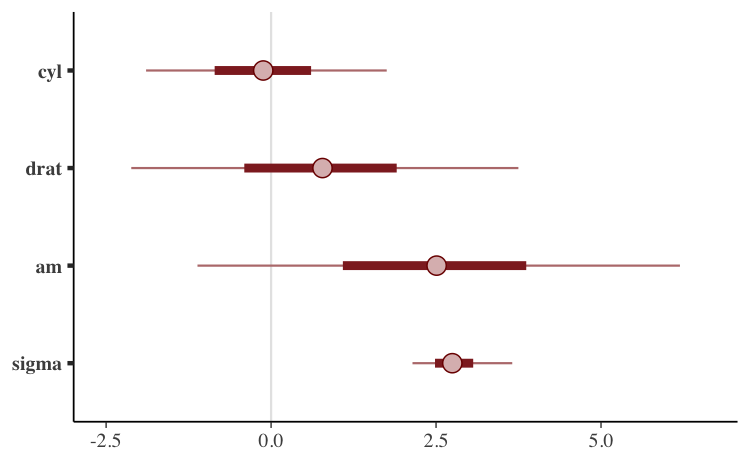The default is to show 50% intervals (the thick segments) and 90% intervals (the thinner outer lines). These defaults can be changed using the `prob` and `prob_outer` arguments, respectively. The points in the above plot are posterior medians. The `point_est` argument can be used to select posterior means instead or to omit the point estimates.

To show the uncertainty intervals as shaded areas under the estimated posterior density curves we can use the `mcmc_areas` function.

``````mcmc_areas(
posterior,
pars = c("cyl", "drat", "am", "sigma"),
prob = 0.8, # 80% intervals
prob_outer = 0.99, # 99%
point_est = "mean"
)``````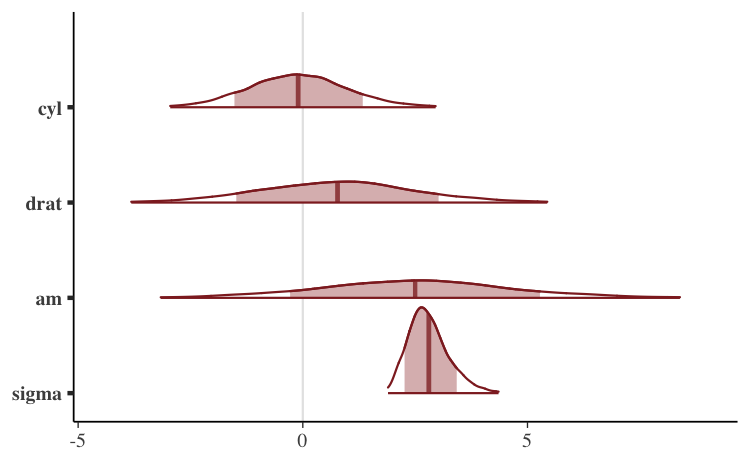## Univariate marginal posterior distributions

bayesplot provides functions for looking at histograms or kernel density estimates of marginal posterior distributions, either with all Markov chains combined or with the chains separate.

Documentation:

#### mcmc_hist

The `mcmc_hist` function plots marginal posterior distributions (combining all chains):

``````color_scheme_set("green")
mcmc_hist(posterior, pars = c("wt", "sigma"))``````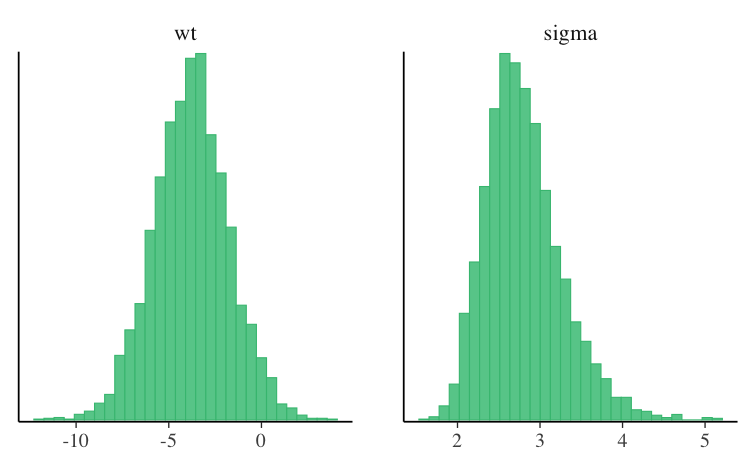If we want to plot `log(sigma)` rather than `sigma` we can either transform the draws in advance or use the `transformations` argument.

``````color_scheme_set("blue")
mcmc_hist(posterior, pars = c("wt", "sigma"),
transformations = list("sigma" = "log"))``````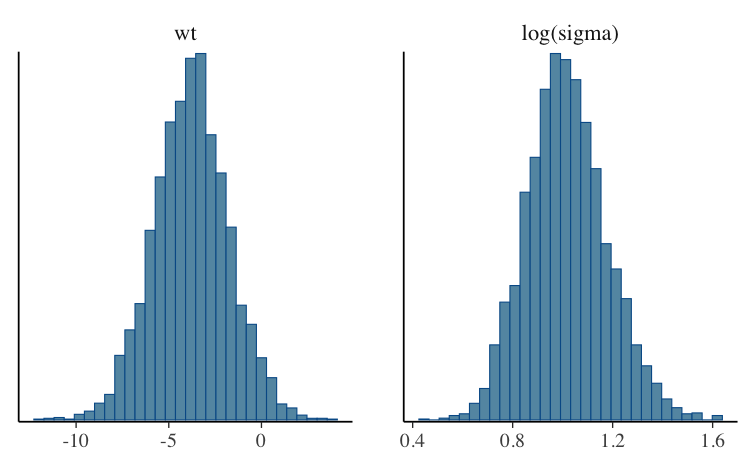Most of the other functions for plotting MCMC draws also have a `transformations` argument.

#### mcmc_hist_by_chain

To view separate histograms of each of the four Markov chains we can use `mcmc_hist_by_chain`, which plots each chain in a separate facet in the plot.

``````color_scheme_set("brightblue")
mcmc_hist_by_chain(posterior, pars = c("wt", "sigma"))``````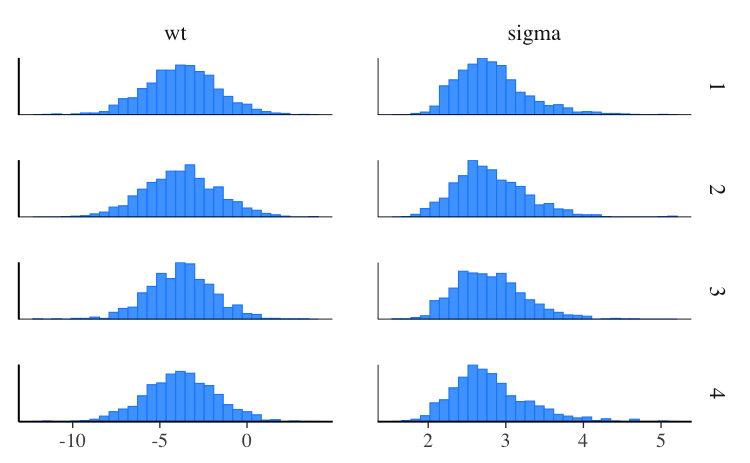#### mcmc_dens

The `mcmc_dens` function is similar to `mcmc_hist` but plots kernel density estimates instead of histograms.

``````color_scheme_set("purple")
mcmc_dens(posterior, pars = c("wt", "sigma"))``````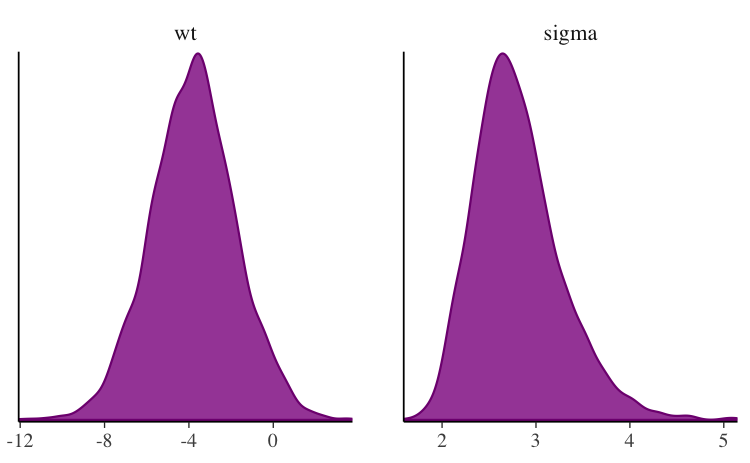#### mcmc_dens_overlay

Like `mcmc_hist_by_chain`, the `mcmc_dens_overlay` function separates the Markov chains. But instead of plotting each chain individually, the density estimates are overlaid.

``mcmc_dens_overlay(posterior, pars = c("wt", "sigma"))``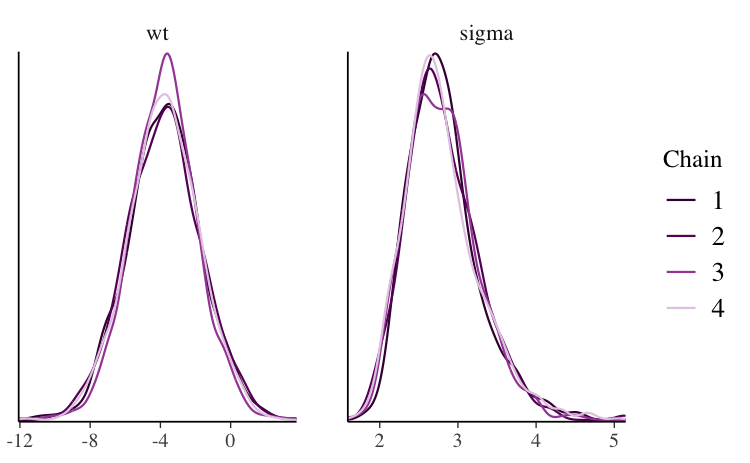#### mcmc_violin

The `mcmc_violin` function plots the density estimates of each chain as violins and draws horizontal line segments at user-specified quantiles.

``````color_scheme_set("teal")
mcmc_violin(posterior, pars = c("wt", "sigma"), probs = c(0.1, 0.5, 0.9))``````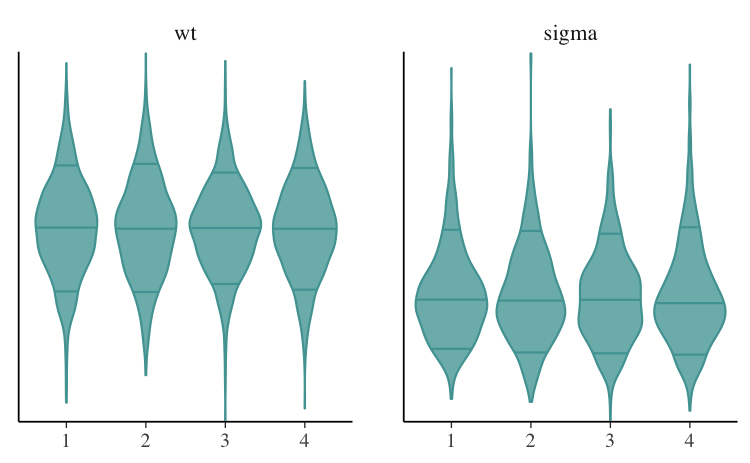## Bivariate plots

Various functions are available for plotting bivariate marginal posterior distributions. Some of these functions also take optional arguments for adding MCMC diagnostic information to the plots. That additional functionality is discussed in the separate Visual MCMC diagnostics vignette.

Documentation:

#### mcmc_scatter

The `mcmc_scatter` function creates a simple scatterplot of two parameters.

``````color_scheme_set("gray")
mcmc_scatter(posterior, pars = c("(Intercept)", "wt"),
size = 1.5, alpha = 0.5)``````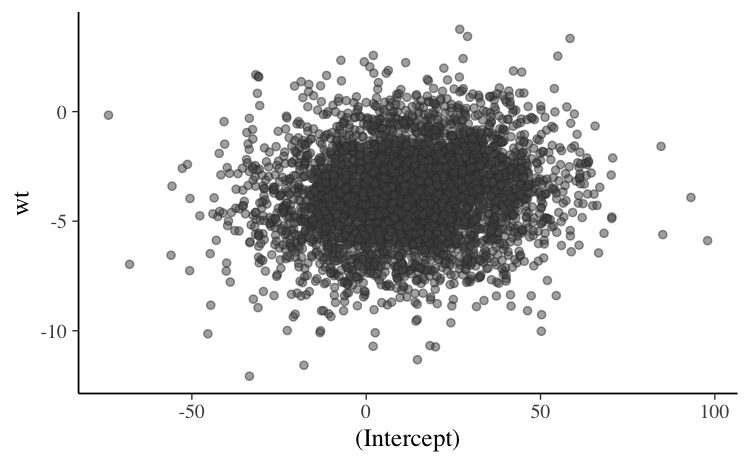#### mcmc_hex

The `mcmc_hex` function creates a similar plot but using hexagonal binning, which can be useful to avoid overplotting.

``````# requires hexbin package
if (requireNamespace("hexbin", quietly = TRUE)) {
mcmc_hex(posterior, pars = c("(Intercept)", "wt"))
}``````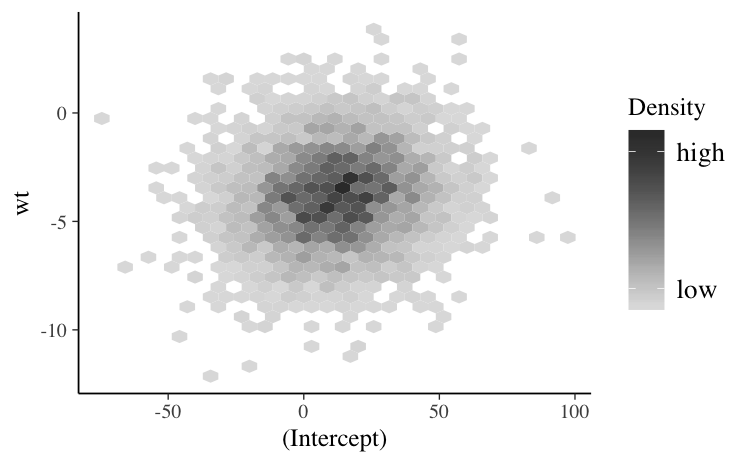#### mcmc_pairs

In addition to `mcmc_scatter` and `mcmc_hex`, bayesplot now provides an `mcmc_pairs` function for creating pairs plots with more than two parameters.

``````color_scheme_set("pink")
mcmc_pairs(posterior, pars = c("(Intercept)", "wt", "sigma"),
off_diag_args = list(size = 1.5))``````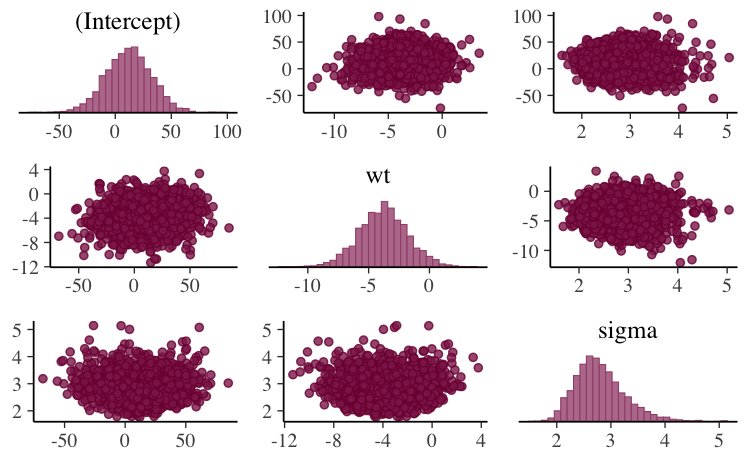The univariate marginal posteriors are shown along the diagonal as histograms, but this can be changed to densities by setting `diag_fun="dens"`. Bivariate plots are displayed above and below the diagonal as scatterplots, but it is also possible to use hex plots by setting `off_diag_fun="hex"`. By default, `mcmc_pairs` shows some of the Markov chains (half, if an even number of chains) above the diagonal and the others below. There are many more options for controlling how the draws should be split between the plots above and below the diagonal (see the documentation for the `condition` argument), but they are more useful when MCMC diagnostic information is included. This is discussed in the Visual MCMC diagnostics vignette.

## Trace plots

Trace plots are time series plots of Markov chains. In this vignette we show the standard trace plots that bayesplot can make. For models fit using any Stan interface (or Hamiltonian Monte Carlo in general), the Visual MCMC diagnostics vignette provides an example of also adding information about divergences to trace plots.

Documentation:

#### mcmc_trace

The `mcmc_trace` function creates standard trace plots:

``````color_scheme_set("blue")
mcmc_trace(posterior, pars = c("wt", "sigma"))``````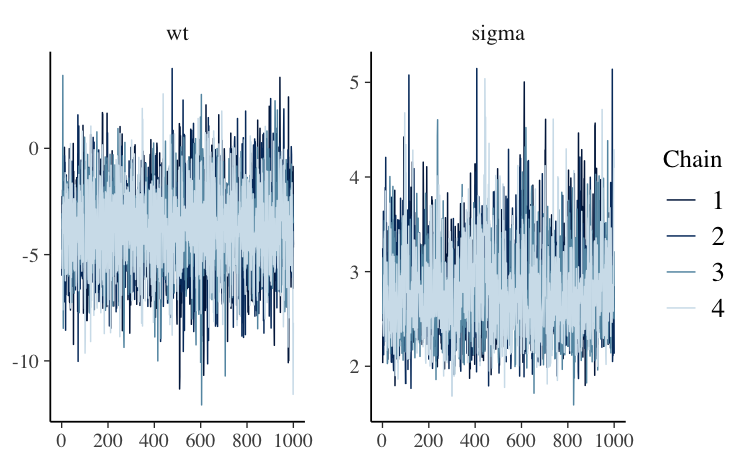If it’s hard to see the difference between the chains we can change to a mixed color scheme, for example:

``````color_scheme_set("mix-blue-red")
mcmc_trace(posterior, pars = c("wt", "sigma"),
facet_args = list(ncol = 1, strip.position = "left"))``````